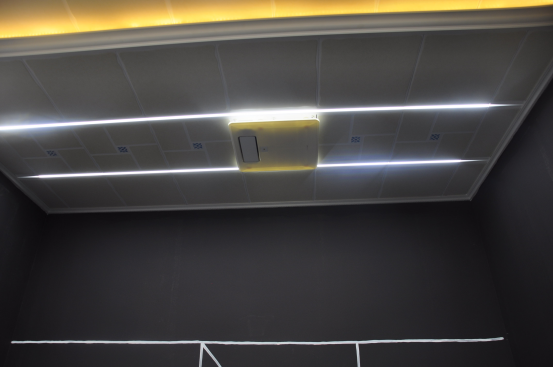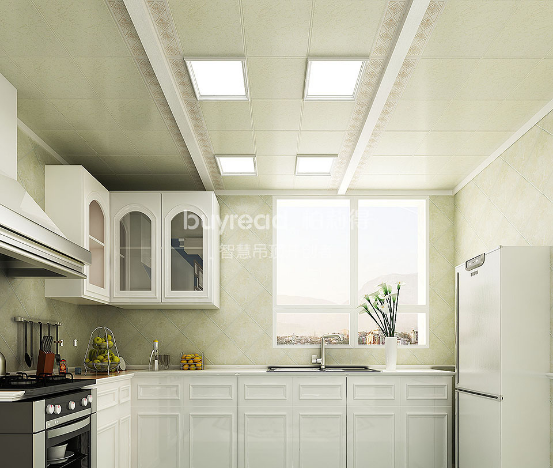|

# 沈佳尉谈智慧吊顶:柏莉得先一步,行业慢半拍!

关于柏莉得智慧吊顶的创始人沈佳尉，有人说他是幻想家，天马行空的想象把智慧吊顶当作漫天驰骋的舞台;有人说他是科技控，一切产品的研发、设计都是基于大数据;也有人说他，在吊顶行业走得有些偏，但他自信地表示：“柏莉得不是走偏，只是行业慢了半拍!”但不管如何柏莉得智慧吊顶在今年的中国建博会(广州)上实实在在地火了一把，至于是不是走偏了方向，让我们一起走进沈佳尉以及他的智慧吊顶世界里一探究竟!

智慧吊顶是什么一个概念，和智能有何区别?

沈总：智慧高于智能，我们的产品更多是带有自动化的功能，不是简单的遥控，不是简单地通过APP来控制。如果只是APP控制或者加个智能化的开关，那么这个技术太LOW了，在几年前就已经实现了，我们要实现的是一连串的反应，产品与产品之前有一个交互。一个产品的使用能够触发另一个产品的功能变化，就像有一个大脑能够自动思考，像拥有了智慧一样，而不只是控制开关!

智慧吊顶的大脑是不是云?

沈总：云更多的只是一个载体，真正的核心在于大数据的运算和处理，云只是一个平台。我们站在云这个平台上，掌控整个家庭的一个状况，数据反馈之后我们经过大数据的处理，来触发其他电器的反应。就像谷歌的超级计算机阿法狗对阵韩国棋手李世石一样，只是我们计算机没有达到谷歌超级计算机的水平，但实际上也是一个人工智能。柏莉得智慧生活可以实现哪些互联?

沈总：目前已经物联了很多的家庭电器，在厨房有智慧化的换气扇，换气扇可以触发油烟机、厨房的窗帘、煤气自动阀门、烟雾报警装置等，如果厨房有可燃性气体，换气扇检测到之后，窗就会自动打开、煤气的阀门会自动关闭。

柏莉得在中国建博会(广州)上展示了哪些新品?有何优势?

沈总：具体有vane系列浴霸、vane系列换气扇、净水器、电动窗帘(与灯智慧一体化)、幕布系统(幕布降下来，灯光会自动调暗，从而进入家庭影院的模式)，按照传统需要一项一项通过开关打开，现在是一连串的自动化。还有空气净化器，在手机端就可以知道家里的空气状况，进行调节空气质量;还有全新的照明系统，可以自动变亮，双色可调节，跟进夜灯模式正白变暖白。

所有的产品都是源自于需求、根据生活实际。

特别是vane系列智慧化浴霸，可检测整个空间的状况，比如温度、湿度、PM值等，更多重要的是可以检测到人在洗澡时有没有摔倒。当下中国已经进入了老龄化社会，国家层面已经重视老年人的生活起居，我们也是基于此开发这款浴霸。老年人摔倒之后往往会引起中风，躺在地上动不了，我们通过热成像传感器，通过手机APP短信推送至亲属那里。未来智慧化吊顶会与智慧化小区进行串联，如果真的有老人摔倒了，我们的浴霸在三分钟之内触发警报，同步告知家属与物业，物业会第一时间到达业主家对老人进行救护，甚至与社区医院进行串联。这是一个庞大的体系，柏莉得智慧吊顶只是这个体系里的一份子，而且我们比行业先做了，到时整个系统搭建完毕，我们只需要直接互联即可。

此外，我们可以精准地知道我们的换气扇风轮转了多少圈，因为风轮转到几十亿转的时候上面就沾满了灰尘，而灰尘会产生静电，对电器的电机造成很多的干扰，我们希望的电机养护方面也是领先行业的。我们提供养护服务，是通过传感器触发告知客户需要保养了，就像我们上4S店保养汽车一样。智慧化社区什么时候才能实现?

沈总：如果有做智慧社区的科技能够与柏莉得合作，最快半年之后就可以实现了。当然最希望更多的大公司一起进入进来完成这件事，把智能家居的产业往前推。

柏莉得的未来目标是什么?

沈总：我们是从传统的制造型企业过来，但目前智慧化时代，再投入更多传统的东西意义不大。柏莉得从传统的制造型企业向科技型企业转变，我们不仅线下有门店，同时在线上推动整个营销，线上引流线下销售。此外门店形象、品牌形象也将进行调整，今后门店将以智慧吊顶为依托，明年可能是智慧吊顶+咖啡，2018年可能就是智能家居+咖啡，不单单是一个集成吊顶门店，还可以是一个咖啡馆，更是设计师创业的一个点。客户要的是装在家里的，而不是展示在店里的，所以我们的展馆跟其他品牌的展馆有很大的不同，没有太多的产品，因为产品可以通过软件和工具进行展示。

柏莉得是与众不同还是走偏了呢?

沈总：目前行业都在往集成墙面走，大家目前并不十分重视智慧化吊顶，但是大家都不重视的东西我也才机会切入。现在我们走在行业前面，走了一条与别人不一样的道路。一旦今后整个行业追上来了，或者发展到一定程度，可以与柏莉得的智慧化家居产品互联了，我们就直接对接即可。你可以说是柏莉得与众不同，而不是柏莉得走偏了，是行业落后于我们半拍!

`声明：本文由入驻焦点开放平台的作者撰写，除焦点官方账号外，观点仅代表作者本人，不代表焦点立场错误信息举报电话： 400-099-0099，邮箱：jubao@vip.sohu.com，或点此进行意见反馈，或点此进行举报投诉。`A B C D E F G H J K L M N P Q R S T W X Y Z
A - B - C - D - E
• A
• 鞍山
• 安庆
• 安阳
• 安顺
• 安康
• 澳门
• B
• 北京
• 保定
• 包头
• 巴彦淖尔
• 本溪
• 蚌埠
• 亳州
• 滨州
• 北海
• 百色
• 巴中
• 毕节
• 保山
• 宝鸡
• 白银
• 巴州
• C
• 承德
• 沧州
• 长治
• 赤峰
• 朝阳
• 长春
• 常州
• 滁州
• 池州
• 长沙
• 常德
• 郴州
• 潮州
• 崇左
• 重庆
• 成都
• 楚雄
• 昌都
• 慈溪
• 常熟
• D
• 大同
• 大连
• 丹东
• 大庆
• 东营
• 德州
• 东莞
• 德阳
• 达州
• 大理
• 德宏
• 定西
• 儋州
• 东平
• E
• 鄂尔多斯
• 鄂州
• 恩施
F - G - H - I - J
• F
• 抚顺
• 阜新
• 阜阳
• 福州
• 抚州
• 佛山
• 防城港
• G
• 赣州
• 广州
• 桂林
• 贵港
• 广元
• 广安
• 贵阳
• 固原
• H
• 邯郸
• 衡水
• 呼和浩特
• 呼伦贝尔
• 葫芦岛
• 哈尔滨
• 黑河
• 淮安
• 杭州
• 湖州
• 合肥
• 淮南
• 淮北
• 黄山
• 菏泽
• 鹤壁
• 黄石
• 黄冈
• 衡阳
• 怀化
• 惠州
• 河源
• 贺州
• 河池
• 海口
• 红河
• 汉中
• 海东
• I
• J
• 晋中
• 锦州
• 吉林
• 鸡西
• 佳木斯
• 嘉兴
• 金华
• 景德镇
• 九江
• 吉安
• 济南
• 济宁
• 焦作
• 荆门
• 荆州
• 江门
• 揭阳
• 金昌
• 酒泉
• 嘉峪关
K - L - M - N - P
• K
• 开封
• 昆明
• 昆山
• L
• 廊坊
• 临汾
• 辽阳
• 连云港
• 丽水
• 六安
• 龙岩
• 莱芜
• 临沂
• 聊城
• 洛阳
• 漯河
• 娄底
• 柳州
• 来宾
• 泸州
• 乐山
• 六盘水
• 丽江
• 临沧
• 拉萨
• 林芝
• 兰州
• 陇南
• M
• 牡丹江
• 马鞍山
• 茂名
• 梅州
• 绵阳
• 眉山
• N
• 南京
• 南通
• 宁波
• 南平
• 宁德
• 南昌
• 南阳
• 南宁
• 内江
• 南充
• P
• 盘锦
• 莆田
• 平顶山
• 濮阳
• 攀枝花
• 普洱
• 平凉
Q - R - S - T - W
• Q
• 秦皇岛
• 齐齐哈尔
• 衢州
• 泉州
• 青岛
• 清远
• 钦州
• 黔南
• 曲靖
• 庆阳
• R
• 日照
• 日喀则
• S
• 石家庄
• 沈阳
• 双鸭山
• 绥化
• 上海
• 苏州
• 宿迁
• 绍兴
• 宿州
• 三明
• 上饶
• 三门峡
• 商丘
• 十堰
• 随州
• 邵阳
• 韶关
• 深圳
• 汕头
• 汕尾
• 三亚
• 三沙
• 遂宁
• 山南
• 商洛
• 石嘴山
• T
• 天津
• 唐山
• 太原
• 通辽
• 铁岭
• 泰州
• 台州
• 铜陵
• 泰安
• 铜仁
• 铜川
• 天水
• 天门
• W
• 乌海
• 乌兰察布
• 无锡
• 温州
• 芜湖
• 潍坊
• 威海
• 武汉
• 梧州
• 渭南
• 武威
• 吴忠
• 乌鲁木齐
X - Y - Z
• X
• 邢台
• 徐州
• 宣城
• 厦门
• 新乡
• 许昌
• 信阳
• 襄阳
• 孝感
• 咸宁
• 湘潭
• 湘西
• 西双版纳
• 西安
• 咸阳
• 西宁
• 仙桃
• 西昌
• Y
• 运城
• 营口
• 盐城
• 扬州
• 鹰潭
• 宜春
• 烟台
• 宜昌
• 岳阳
• 益阳
• 永州
• 阳江
• 云浮
• 玉林
• 宜宾
• 雅安
• 玉溪
• 延安
• 榆林
• 银川
• Z
• 张家口
• 镇江
• 舟山
• 漳州
• 淄博
• 枣庄
• 郑州
• 周口
• 驻马店
• 株洲
• 张家界
• 珠海
• 湛江
• 肇庆
• 中山
• 自贡
• 资阳
• 遵义
• 昭通
• 张掖
• 中卫

1室1厅1厨1卫1阳台

1
2
3
4
5

0
1
2

1

1

0
1
2
3报名成功，资料已提交审核A B C D E F G H J K L M N P Q R S T W X Y Z
A - B - C - D - E
• A
• 鞍山
• 安庆
• 安阳
• 安顺
• 安康
• 澳门
• B
• 北京
• 保定
• 包头
• 巴彦淖尔
• 本溪
• 蚌埠
• 亳州
• 滨州
• 北海
• 百色
• 巴中
• 毕节
• 保山
• 宝鸡
• 白银
• 巴州
• C
• 承德
• 沧州
• 长治
• 赤峰
• 朝阳
• 长春
• 常州
• 滁州
• 池州
• 长沙
• 常德
• 郴州
• 潮州
• 崇左
• 重庆
• 成都
• 楚雄
• 昌都
• 慈溪
• 常熟
• D
• 大同
• 大连
• 丹东
• 大庆
• 东营
• 德州
• 东莞
• 德阳
• 达州
• 大理
• 德宏
• 定西
• 儋州
• 东平
• E
• 鄂尔多斯
• 鄂州
• 恩施
F - G - H - I - J
• F
• 抚顺
• 阜新
• 阜阳
• 福州
• 抚州
• 佛山
• 防城港
• G
• 赣州
• 广州
• 桂林
• 贵港
• 广元
• 广安
• 贵阳
• 固原
• H
• 邯郸
• 衡水
• 呼和浩特
• 呼伦贝尔
• 葫芦岛
• 哈尔滨
• 黑河
• 淮安
• 杭州
• 湖州
• 合肥
• 淮南
• 淮北
• 黄山
• 菏泽
• 鹤壁
• 黄石
• 黄冈
• 衡阳
• 怀化
• 惠州
• 河源
• 贺州
• 河池
• 海口
• 红河
• 汉中
• 海东
• I
• J
• 晋中
• 锦州
• 吉林
• 鸡西
• 佳木斯
• 嘉兴
• 金华
• 景德镇
• 九江
• 吉安
• 济南
• 济宁
• 焦作
• 荆门
• 荆州
• 江门
• 揭阳
• 金昌
• 酒泉
• 嘉峪关
K - L - M - N - P
• K
• 开封
• 昆明
• 昆山
• L
• 廊坊
• 临汾
• 辽阳
• 连云港
• 丽水
• 六安
• 龙岩
• 莱芜
• 临沂
• 聊城
• 洛阳
• 漯河
• 娄底
• 柳州
• 来宾
• 泸州
• 乐山
• 六盘水
• 丽江
• 临沧
• 拉萨
• 林芝
• 兰州
• 陇南
• M
• 牡丹江
• 马鞍山
• 茂名
• 梅州
• 绵阳
• 眉山
• N
• 南京
• 南通
• 宁波
• 南平
• 宁德
• 南昌
• 南阳
• 南宁
• 内江
• 南充
• P
• 盘锦
• 莆田
• 平顶山
• 濮阳
• 攀枝花
• 普洱
• 平凉
Q - R - S - T - W
• Q
• 秦皇岛
• 齐齐哈尔
• 衢州
• 泉州
• 青岛
• 清远
• 钦州
• 黔南
• 曲靖
• 庆阳
• R
• 日照
• 日喀则
• S
• 石家庄
• 沈阳
• 双鸭山
• 绥化
• 上海
• 苏州
• 宿迁
• 绍兴
• 宿州
• 三明
• 上饶
• 三门峡
• 商丘
• 十堰
• 随州
• 邵阳
• 韶关
• 深圳
• 汕头
• 汕尾
• 三亚
• 三沙
• 遂宁
• 山南
• 商洛
• 石嘴山
• T
• 天津
• 唐山
• 太原
• 通辽
• 铁岭
• 泰州
• 台州
• 铜陵
• 泰安
• 铜仁
• 铜川
• 天水
• 天门
• W
• 乌海
• 乌兰察布
• 无锡
• 温州
• 芜湖
• 潍坊
• 威海
• 武汉
• 梧州
• 渭南
• 武威
• 吴忠
• 乌鲁木齐
X - Y - Z
• X
• 邢台
• 徐州
• 宣城
• 厦门
• 新乡
• 许昌
• 信阳
• 襄阳
• 孝感
• 咸宁
• 湘潭
• 湘西
• 西双版纳
• 西安
• 咸阳
• 西宁
• 仙桃
• 西昌
• Y
• 运城
• 营口
• 盐城
• 扬州
• 鹰潭
• 宜春
• 烟台
• 宜昌
• 岳阳
• 益阳
• 永州
• 阳江
• 云浮
• 玉林
• 宜宾
• 雅安
• 玉溪
• 延安
• 榆林
• 银川
• Z
• 张家口
• 镇江
• 舟山
• 漳州
• 淄博
• 枣庄
• 郑州
• 周口
• 驻马店
• 株洲
• 张家界
• 珠海
• 湛江
• 肇庆
• 中山
• 自贡
• 资阳
• 遵义
• 昭通
• 张掖
• 中卫• 手机• 分享
• 设计
免费设计
• 计算器
装修计算器
• 入驻
合作入驻
• 联系
联系我们
• 置顶
返回顶部